## Implicit Suggestions

One of the unexpected and rewarding aspects of having started this blog is being contacted out of the blue by students. This included an extended correspondence with one particular VCE student, whom we have never met and of whom we know very little, other than that this year they undertook UMEP mathematics (Melbourne University extension). The student emailed again recently, about the final question on this year’s (calculator-free) Specialist Mathematics Exam 1 (not online). Though perhaps not (but also perhaps yes) a WitCH, the exam question (below), and the student’s comments (belower), seemed worth sharing.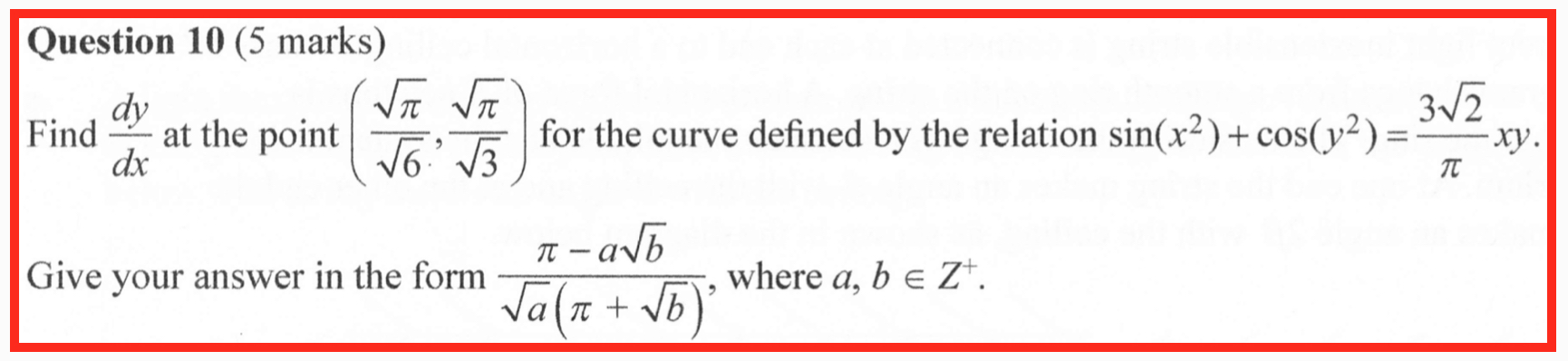Hi Marty,

Have a peek at Question 10 of Specialist 2019 Exam 1 when you get a chance. It was a 5 mark question, only roughly 2 of which actually assessed relevant Specialist knowledge – the rest was mechanical manipulation of ugly fractions and surds. Whilst I happened to get the right answer, I know of talented others who didn’t.

I saw a comment you made on the blog regarding timing sometime recently, and I couldn’t agree more. I made more stupid mistakes than I would’ve liked on the Specialist exam 2, being under pressure to race against the clock. It seems honestly pathetic to me that VCAA can only seem to differentiate students by time. (Especially when giving 2 1/2 hours for science subjects, with no reason why they can’t do the same for Maths.) It truly seems a pathetic way to assess or distinguish between proper mathematical talent and button-pushing speed writing.

I definitely appreciate the UMEP exams. We have 3 hrs and no CAS! That, coupled with the assignments that expect justification and insight, certainly makes me appreciate maths significantly more than from VCE. My only regret on that note was that I couldn’t do two UMEP subjects 🙂

## A Loss of Momentum

The VCE maths exams are over for another year. They were mostly uneventful, the familiar concoction of triviality, nonsense and weirdness, with the notable exception of the surprisingly good Methods Exam 1. At least two Specialist questions, however, deserve a specific slap and some discussion. (There may be other questions worth whacking: we never have the stomach to give VCE exams a close read.)

Question 6 on Specialist Exam 1 concerns a particle acted on by a force, and students are asked to

Find the change in momentum in kg ms-2 …

Doh!

The problem of course is that the suggested units are for force rather than momentum. This is a straight-out error and there’s not much to be said (though see below).

Then there’s Question 3 on part 2 of Specialist Exam 2. This question is concerned with a fountain, with water flowing in from a jet and flowing out at the bottom. The fountaining is distractingly irrelevant, reminiscent of a non-flying bee, but we have larger concerns.

In part (c)(i) of the question students are required to show that the height h of the water in the fountain is governed by the differential equation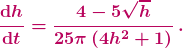The problem is with the final part (f) of the question, where students are asked

How far from the top of the fountain does the water level ultimately stabilise?

The question is typical in its clumsy and opaque wording. One could have asked more simply for the depth h of the water, which would at least have cleared the way for students to consider the true weirdness of the question: what is meant by “ultimately stabilise”?

The examiners are presumably expecting students to set dh/dt = 0, to obtain the constant, equilibrium solution (and then to subtract the equilibrium value from the height of the fountain because why not give students the opportunity to blow half their marks by misreading a convoluted question?) The first problem with that is, as we have pointed out before, equilibria of differential equations appear nowhere in the Specialist curriculum. The second problem is, as we have pointed out before, not all equilibria are stable.

It would be smart and good if the VCAA decided to include equilibrium solutions in the Specialist curriculum, along with some reasonable analysis and application. Until they do, however, questions such as the above are unfair and absurd, made all the more unfair and absurd by the inevitably awful wording.

********************

Now, what to make of these two questions? How much should VCAA be hammered?

We’re not so concerned about the momentum error. It is unfortunate, it would have confused many students and it shouldn’t have happened, but a typo is a typo, without deeper meaning.

It appears that Specialist teachers have been less forgiving, and fair enough: the VCAA examiners are notoriously nitpicky, sanctimonious and unapologetic, so they can hardly complain if the same, with greater justification, is done to them. (We also heard of some second-guessing, some suggestions that the units of “change in momentum” could be or are the same as the units of force. This has to be Stockholm syndrome.)

The fountain question is of much greater concern because it exemplifies systemic issues with the curriculum and the manner in which it is examined. Above all, assessment must be fair and reasonable, which means students and teachers must be clearly told what is examinable and how it may be examined. As it stands, that is simply not the case, for either Specialist or Methods.

Notably, however, we have heard of essentially no complaints from Specialist teachers regarding the fountain question; just one teacher pointed out the issue to us. Undoubtedly there were other teachers bothered by the question, but the relative silence in comparison to the vocal complaints on the momentum typo is stark. And unfortunate.

There is undoubted satisfaction in nitpicking the VCAA in a sauce for the goose manner. But a typo is a typo, and teachers shouldn’t engage in small-time point-scoring any more than VCAA examiners.

The real issue is that the current curriculum is shallow, aimless, clunky, calculator-poisoned, effectively undefined and effectively unexaminable. All of that matters infinitely more than one careless mistake.

## Update (24/02/19)

The exam Reports are now out, here and here. There’s no stupidity so large or so small that the VCAA won’t remain silent.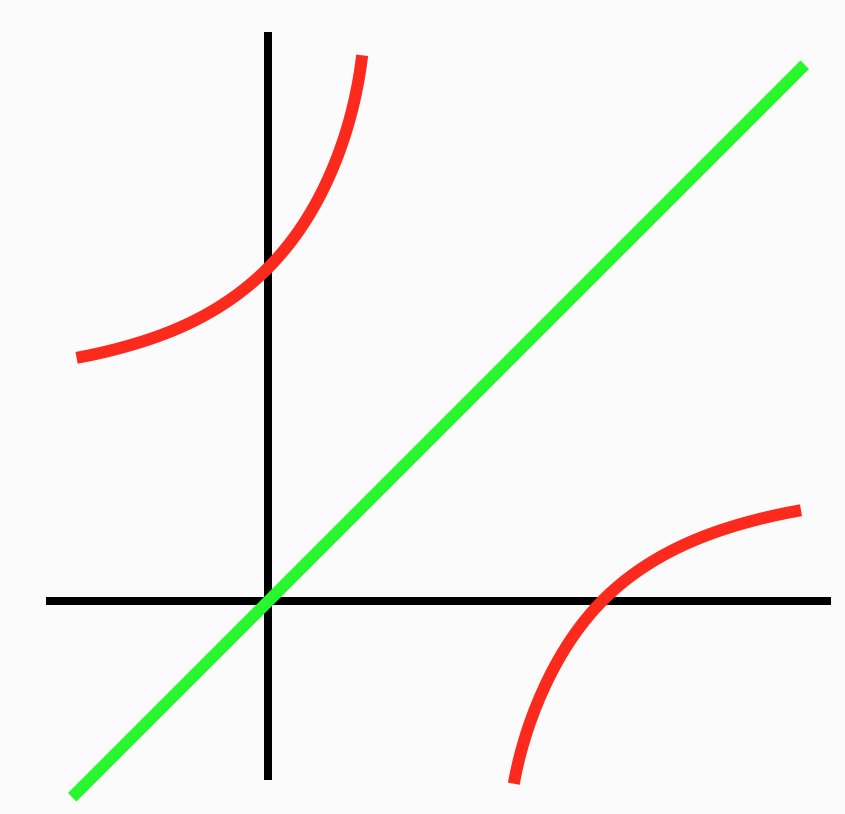Our sixth and final post on the 2017 VCE exam madness is on some recurring nonsense in Mathematical Methods. The post will be relatively brief, since a proper critique of every instance of the nonsense would be painfully long, and since we’ve said it all before.

The mathematical problem concerns, for a given function f, finding the solutions to the equation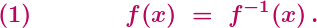This problem appeared, in various contexts, on last month’s Exam 2 in 2017 (Section B, Questions 4(c) and 4(i)), on the Northern Hemisphere Exam 1 in 2017 (Questions 8(b) and 8(c)), on Exam 2 in 2011 (Section 2, Question 3(c)(ii)), and on Exam 2 in 2010 (Section 2, Question 1(a)(iii)).

Unfortunately, the technique presented in the three Examiners’ Reports for solving equation (1) is fundamentally wrong. (The Reports are here, here and here.) In synch with this wrongness, the standard textbook considers four misleading examples, and its treatment of the examples is infused with wrongness (Chapter 1F). It’s a safe bet that the forthcoming Report on the 2017 Methods Exam 2 will be plenty wrong.

What is the promoted technique? It is to ignore the difficult equation above, and to solve instead the presumably simpler equationor perhaps the equationWhich is wrong.

It is simply not valid to assume that either equation (2) or (2)’ is equivalent to (1). Yes, as long as the inverse of f exists then equation (2)’ is equivalent to equation (2): a solution x to (2)’ will also be a solution to (2), and vice versa. And, yes, then any solution to (2) and (2)’ will also be a solution to (1). The converse, however, is in general false: a solution to (1) need not be a solution to (2) or (2)’.

It is easy to come up with functions illustrating this, or think about the graph above, or look here.

OK, the VCAA might argue that the exams (and, except for a couple of up-in-the-attic exercises, the textbook) are always concerned with functions for which solving (2) or (2)’ happens to suffice, so what’s the problem? The problem is that this argument would be idiotic.

Suppose that we taught students that roots of polynomials are always integers, instructed the students to only check for integer solutions, and then carefully arranged for the students to only encounter polynomials with integer solutions. Clearly, that would be mathematical and pedagogical crap. The treatment of equation (1) in Methods exams, and the close to universal treatment in Methods more generally, is identical.

OK, the VCAA might continue to argue that the students have their (stupifying) CAS machines at hand, and that the graphs of the particular functions under consideration make clear that solving (2) or (2)’ suffices. There would then be three responses:

(i) No one tests whether Methods students do anything like a graphical check, or anything whatsoever.

(ii) Hardly any Methods students do do anything. The overwhelming majority of students treat equations (1), (2) and (2)’ as automatically equivalent, and they have been given explicit license by the Examiners’ Reports to do so. Teachers know this and the VCAA knows this, and any claim otherwise is a blatant lie. And, for any reader still in doubt about what Methods students actually do, here’s a thought experiment: imagine the 2018 Methods exam requires students to solve equation (1) for the function f(x) = (x-2)/(x-1), and then imagine the consequences.

(iii) Even if students were implicitly or explicitly arguing from CAS graphics, “Look at the picture” is an absurdly impoverished way to think about or to teach mathematics, or pretty much anything. The power of mathematics is to be able take the intuition and to either demonstrate what appears to be true, or demonstrate that the intuition is misleading. Wise people are wary of the treachery of images; the VCAA, alas, promotes it.

The real irony and idiocy of this situation is that, with natural conditions on the function f, equation (1) is equivalent to equations (2) and (2)’, and that it is well within reach of Methods students to prove this. If, for example, f is a strictly increasing function then it can readily be proved that the three equations are equivalent. Working through and applying such results would make for excellent lessons and excellent exam questions.

Instead, what we have is crap. Every year, year after year, thousands of Methods students are being taught and are being tested on mathematical crap.

## The Madness of Crowd Models

Our fifth and penultimate post on the 2017 VCE exam madness concerns Question 3 of Section B on the Northern Hemisphere Specialist Mathematics Exam 2. The question begins with the logistic equation for the proportion P of a petri dish covered by bacteria: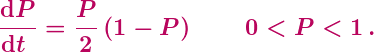This is not a great start, since it’s a little peculiar using the logistic equation to model an area proportion, rather than a population or a population density. It’s also worth noting that the strict inequalities on P are unnecessary and rule out of consideration the equilibrium (constant) solutions P = 0 and P = 1.

Clunky framing aside, part (a) of Question 3 is pretty standard, requiring the solving of the above (separable) differential equation with initial condition P(0) = 1/2. So, a decent integration problem trivialised by the presence of the stupifying CAS machine. After which things go seriously off the rails.

The setting for part (b) of the question has a toxin added to the petri dish at time t = 1, with the bacterial growth then modelled by the equation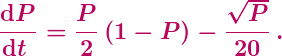Well, probably not. The effect of toxins is most simply modelled as depending linearly on P, and there seems to be no argument for the square root. Still, this kind of fantasy modelling is par for the VCAA‘s crazy course. Then, however, comes Question 3(b):

Find the limiting value of P, which is the maximum possible proportion of the Petri dish that can now be covered by the bacteria.

The question is a mess. And it’s wrong.

The Examiners’ “Report” (which is not a report at all, but merely a list of short answers) fails to indicate what students did or how well they did on this short, 2-mark question. Presumably the intent was for students to find the limit of P by finding the maximal equilibrium solution of the differential equation. So, setting dP/dt = 0 implies that the right hand side of the differential equation is also 0. The resulting equation is not particularly nice, a quartic equation for Q = √P. Just more silly CAS stuff, then, giving the largest solution P = 0.894 to the requested three decimal places.

In principle, applying that approach here is fine. There are, however, two major problems.

The first problem is with the wording of the question: “maximum possible proportion” simply does not mean maximal equilibrium solution, nor much of anything. The maximum possible proportion covered by the bacteria is P = 1. Alternatively, if we follow the examiners and needlessly exclude = 1 from consideration, then there is no maximum possible proportion, and P can just be arbitrarily close to 1. Either way, a large initial P will decay down to the maximal equilibrium solution.

One might argue that the examiners had in mind a continuation of part (a), so that the proportion begins below the equilibrium value and then rises towards it. That wouldn’t rescue the wording, however. The equilibrium solution is still not a maximum, since the equilibrium value is never actually attained. The expression the examiners are missing, and possibly may even have heard of, is least upper bound. That expression is too sophisticated to be used on a school exam, but whose problem is that? It’s the examiners who painted themselves into a corner.

The second issue is that it is not at all obvious – indeed it can easily fail to be true – that the maximal equilibrium solution for P will also be the limiting value of P. The garbled information within question (b) is instructing students to simply assume this. Well, ok, it’s their question. But why go to such lengths to impose a dubious and impossible-to-word assumption, rather than simply asking directly for an equilibrium solution?

To clarify the issues here, and to show why the examiners were pretty much doomed to make a mess of things, consider the following differential equation:By setting Q = √P, for example, it is easy to show that the equilibrium solutions are P = 0 and P = 1/4. Moreover, by considering the sign of dP/dt for P above and below the equilibrium P = 1/4, it is easy to obtain a qualitative sense of the general solutions to the differential equation:In particular, it is easy to see that the constant solution P = 1/4 is a semi-stable equilibrium: if P(0) is slightly below 1/4 then P(t) will decay to the stable equilibrium P = 0.

This type of analysis, which can readily be performed on the toxin equation above, is simple, natural and powerful. And, it seems, non-existent in Specialist Mathematics. The curriculum  contains nothing that suggests or promotes any such analysis, nor even a mention of equilibrium solutions. The same holds for the standard textbook, in which for, for example, the equation for Newton’s law of cooling is solved (clumsily), but there’s not a word of insight into the solutions.

And this explains why the examiners were doomed to fail. Yes, they almost stumbled into writing a good, mathematically rich exam question. The paper thin curriculum, however, wouldn’t permit it.

## A Madness for all Seasons

Our fourth post on the  2017 VCE exam madness will be similar to our previous post: a quick whack of a straight-out error. This error was flagged by a teacher friend, David. (No, not that David.)

The 11th multiple choice question on the first Further Mathematics Exam reads as follows:

Which one of the following statistics can never be negative?

A. the maximum value in a data set

B. the value of a Pearson correlation coefficient

C. the value of a moving mean in a smoothed time series

D. the value of a seasonal index

E. the value of a slope of a least squares line fitted to a scatterplot

Before we get started, a quick word on the question’s repeated use of the redundant “the value of”.

Bleah!

Now, on with answering the question.

It is pretty obvious that the statistics in A, B, C and E can all be negative, so presumably the intended answer is D. However, D is also wrong: a seasonal index can also be negative. Unfortunately the explanation of “seasonal index” in the standard textbook is lost in a jungle of non-explanation, so to illustrate we’ll work through a very simple example.

Suppose a company’s profits and losses over the four quarters of a year are as follows:So, the total profit over the year is $8,000, and then the average quarterly profit is$2000. The seasonal index (SI) for each quarter is then that quarter’s profit (or loss) divided by the average quarterly profit:Clearly this example is general, in the sense that in any scenario where the seasonal data are both positive and negative, some of the seasonal indices will be negative. So, the exam question is not merely technically wrong, with a contrived example raising issues: the question is wrong wrong.

Now, to be fair, this time the VCAA has a defense. It appears to be more common to apply seasonal indices in contexts where all the data are one sign, or to use absolute values to then consider magnitudes of deviations. It also appears that most or all examples Further students would have studied included only positive data.

So, yes, the VCAA (and the Australian Curriculum) don’t bother to clarify the definition or permitted contexts for seasonal indices. And yes, the definition in the standard textbook implicitly permits negative seasonal indices. And yes, by this definition the exam question is plain wrong. But, hopefully most students weren’t paying sufficient attention to realise that the VCAA weren’t paying sufficient attention, and so all is ok.

Well, the defense is something like that. The VCAA can work on the wording.

Our third post on the 2017 VCE exam madness will be brief, on a question containing a flagrant error.

The first question in the matrix module of Further Mathematics’ Exam 2 is concerned with a school canteen selling pies, rolls and sandwiches over three separate weeks. The number of items sold is set up as a 3 x 3 matrix, one row for each week and one column for each food choice. The last part, (c)(ii), of the question then reads:

The matrix equation below shows that the total value of all rolls and sandwiches sold in these three weeks is \$915.60Matrix L in this equation is of order 1 x 3.

Write down matrix L.

This 1-mark question is presumably meant to be a gimme, with answer L = [0 1 1]. Unfortunately the question is both weird and wrong. (And lacking in punctuation. Guys, it’s not that hard.) The wrongness comes from the examiners having confused their rows and columns. As is made clear in the the previous part, (c)(i), of the question, the  3 x 1 matrix of numbers indicates the total earnings from each of the three weeks, not from each of the three food choices. So, the equation indicates the total value of all products sold in weeks 2 and 3.

There’s not much to say about such an obvious error. It is very easy to confuse rows and columns, and we’ve all done it on occasion, but if VCAA’s vetting cannot catch this kind of mistake then it cannot be relied upon to catch anything. The only question is how the Examiners’ Report will eventually address the error. The VCAA is well-practised in cowardly silence and weasel-wording, but it would be exceptionally Trumplike to attempt such tactics here.

Error aside, the question is artificial, and it is not clear that the matrix equation “shows” much of anything. Yes, 0-1 and on-or-off matrices are important and useful, but the use of such a matrix in this context is contrived and confusing. Not a hanging offence, and benign by VCAA’s standards, but the question is pretty silly. And, not forgetting, wrong.

Our second post on the 2017 VCE exam madness concerns a question on the first Specialist Mathematics exam. Typically Specialist exams, particularly the first exams, don’t go too far off the rails; it’s usually more “meh” than madness. (Not that “meh” is an overwhelming endorsement of what is nominally a special mathematics subject.) This year, however, the Specialist exams have some notably Methodsy bits. The following nonsense was pointed out to us by John, a friend and colleague.

The final question, Question 10, on the first Specialist exam concerns the function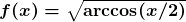, on its maximal domain [-2,2]. In part (c), students are asked to determine the volume of the solid of revolution formed when the region under the graph of f is rotated around the x-axis. This leads to the integralStudents don’t have their stupifying CAS machines in this first exam, so how to do the integral? It is natural to consider integration by parts, but unfortunately this standard and powerful technique is no longer part of the VCE curriculum. (Why not? You’ll have to ask the clowns at ACARA and the VCAA.)

No matter. The VCAA examiners love to have the students to go through a faux-parts computation. So, in part (a) of the question, students are asked to check the derivative of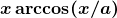. Setting a = 2 in the resulting equation, this givesWe can now integrate and rearrange, giving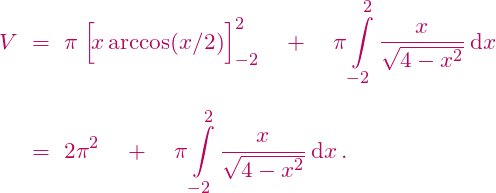So, all that remains is to do that last integral, and … uh oh.

It is easy to integrate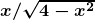indefinitely by substitution, but the problem is that our definite(ish) integral is improper at both endpoints. And, unfortunately, improper integrals are not part of the VCE curriculum. (Why not? You’ll have to ask the clowns at ACARA and the VCAA.) Moreover, even if improper integrals were available, the double improperness is fiddly: we are not permitted to simply integrate from some –b to b and then let b tend to 2.

So, what is a Specialist student to do? One can hope to argue that the integral is zero by odd symmetry, but the improperness is again an issue. As an example indicating the difficulty, the integralis not equal to 0. (The TI Inspire falsely computes the integral to be 0, which is less than inspiring.) Any argument which arrives at the answer 0 for integrating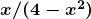is invalid, and is thus prima facie invalid for integratingas well.

Now, in fact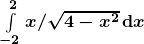is equal to zero, and so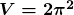. In particular, it is possible to argue that the fatal problem withdoes not occur for our integral, and so both the substitution and symmetry approaches can be made to work. The argument, however, is subtle, well beyond what is expected in a Specialist course.

Note also that this improperness could have been avoided, with no harm to the question, simply by taking the original domain to be, for example, [-1,1]. Which was exactly the approach taken on Question 5 of the 2017 Northern Hemisphere Specialist Exam 1. God knows why it wasn’t done here, but it wasn’t and the consequently the examiners have trouble ahead.

The blunt fact is, Specialist students cannot validly computewith any technique they would have seen in a standard Specialist class. They must either argue incompletely by symmetry or ride roughshod over the improperness. The Examiners’ Report will be a while coming out, though presumably the examiners will accept either argument. But here is a safe prediction: the Report will either contain mealy-mouthed nonsense or blatant mathematical falsehoods. The only alternative is for the examiners to make a clear admission that they stuffed up. Which won’t happen.

Finally, the irony. Look again at the original integral for V. Though this integral arose in the calculation of a volume, it can still be interpreted as the area under the graph of the function y = arccos(x/2):But now we can consider the corresponding area under the inverse function y = 2cos(x):It follows thatDone.

This inverse function trick is standard for Specialist (and Methods) students, and so the students can readily calculate the volume V in this manner. True, reinterpreting the integral for V as an area is a sharp conceptual shift, but with appropriate wording it could have made for a very good Specialist question.

In summary, the Specialist Examiners guided the students to calculate V with a jerry-built technique, leading to an integral that the students cannot validly compute, all the while avoiding a simpler approach well within the students’ grasp. Well played, Examiners, well played.

## There’s Madness in the Methods

Yes, we’ve used that title before, but it’s a damn good title. And there is so much madness in Mathematical Methods to cover. And not only Methods. Victoria’s VCE exams are coming to an end, the maths exams are done, and there is all manner of new and astonishing nonsense to consider. This year, the Victorian Curriculum and Assessment Authority have outdone themselves.

Over the next week we’ll put up a series of posts on significant errors in the 2017 Methods, Specialist Maths and Further Maths exams, including in the mid-year Northern Hemisphere examsBy “significant error” we mean more than just a pointless exercise in button-pushing, or tone-deaf wording, or idiotic pseudomodelling, or aimless pedantry, all of which is endemic in VCE maths exams. A “significant error” in an exam question refers to a fundamental mathematical flaw with the phrasing, or with the intended answer, or with the (presumed or stated) method that students were supposed to use. Not all the errors that we shall discuss are large, but they are all definite errors, they are errors that would have (or at least should have) misled some students, and none of these errors should have occurred. (It is courtesy of diligent (and very annoyed) maths teachers that I learned of most of these questions.) Once we’ve documented the errors, we’ll post on the reasons that the errors are so prevalent, on the pedagogical and administrative climate that permits and encourages them.

Our first post concerns Exam 1 of Mathematical Methods. In the final question, Question 9, students consider the function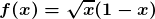on the closed interval [0,1], pictured below. In part (b), students are required to show that, on the open interval (0,1), “the gradient of the tangent to the graph of f” is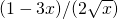. A clumsy combination of calculation and interpretation, but ok. The problem comes when students then have to consider tangents to the graph.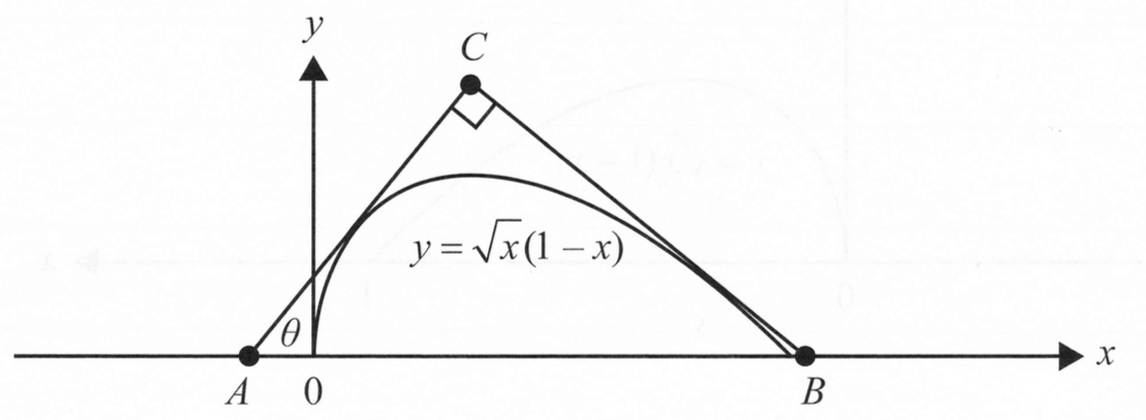In part (c), students take the angle θ in the picture to be 45 degrees. The pictured tangents then have slopes 1 and -1, and the students are required to find the equations of these two tangents. And therein lies the problem: it turns out that the “derivative”  of f is equal to -1 at the endpoint x = 1. However, though the natural domain of the function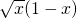is [0,∞), the students are explicitly told that the domain of f is [0,1].

This is obvious and unmitigated madness.

Before we hammer the madness, however, let’s clarify the underlying mathematics.

Does the derivative/tangent of a suitably nice function exist at an endpoint? It depends upon who you ask. If the “derivative” is to exist then the standard “first principles” definition must be modified to be a one-sided limit. So, for our function f above, we would defineThis is clearly not too difficult to do, and with this definition we find that f'(1) = -1, as implied by the Exam question. (Note that since f naturally extends to the right of =1, the actual limit computation can be circumvented.) However, and this is the fundamental point, not everyone does this.

At the university level it is common, though far from universal, to permit differentiability at the endpoints. (The corresponding definition of continuity on a closed interval is essentially universal, at least after first year.) At the school level, however, the waters are much muddier. The VCE curriculum and the most popular and most respected Methods textbook appear to be completely silent on the issue. (This textbook also totally garbles the related issue of derivatives of piecewise defined (“hybrid”) functions.) We suspect that the vast majority of Methods teachers are similarly silent, and that the minority of teachers who do raise the issue would not in general permit differentiability at an endpoint.

In summary, it is perfectly acceptable to permit derivatives/tangents to graphs at their endpoints, and it is perfectly acceptable to proscribe them. It is also perfectly acceptable, at least at the school level, to avoid the issue entirely, as is done in the VCE curriculum, by most teachers and, in particular, in part (b) of the Exam question above.

What is blatantly unacceptable is for the VCAA examiners to spring a completely gratuitous endpoint derivative on students when the issue has never been raised. And what is pure and unadulterated madness is to spring an endpoint derivative after carefully and explicitly avoiding it on the immediately previous part of the question.

The Victorian Curriculum and Assessment Authority has a long tradition of scoring own goals. The question above, however, is spectacular. Here, the VCAA is like a goalkeeper grasping the ball firmly in both hands, taking careful aim, and flinging the ball into his own net.

## The Median is the Message

Our first post concerns an error in the 2016 Mathematical Methods Exam 2 (year 12 in Victoria, Australia). It is not close to the silliest mathematics we’ve come across, and not even the silliest error to occur in a Methods exam. Indeed, most Methods exams are riddled with nonsense. For several reasons, however, whacking this particular error is a good way to begin: the error occurs in a recent and important exam; the error is pretty dumb; it took a special effort to make the error; and the subsequent handling of the error demonstrates the fundamental (lack of) character of the Victorian Curriculum and Assessment Authority.

The problem, first pointed out to us by teacher and friend John Kermond, is in Section B of the exam and concerns Question 3(h)(ii). This question relates to a probability distribution with “probability density function”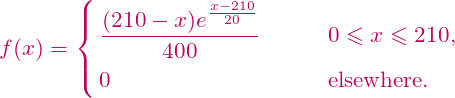Now, anyone with a good nose for calculus is going to be thinking “uh-oh”. It is a fundamental property of a PDF that the total integral (underlying area) should equal 1. But how are all those integrated powers of e going to cancel out? Well, they don’t. What has been defined is only approximately a PDF,  with a total area of. (It is easy to calculate the area exactly using integration by parts.)

Below we’ll discuss the absurdity of handing students a non-PDF, but back to the exam question. 3(h)(ii) asks the students to find the median of the “probability distribution”, correct to two decimal places. Since the question makes no sense for a non-PDF, of course the VCAA have shot themself in the foot. However, we can still attempt to make some sense of the question, which is when we discover that the VCAA has also shot themself in the other foot.

The median m of a probability distribution is the half-way point. So, in the integration context here we want the m for which

a)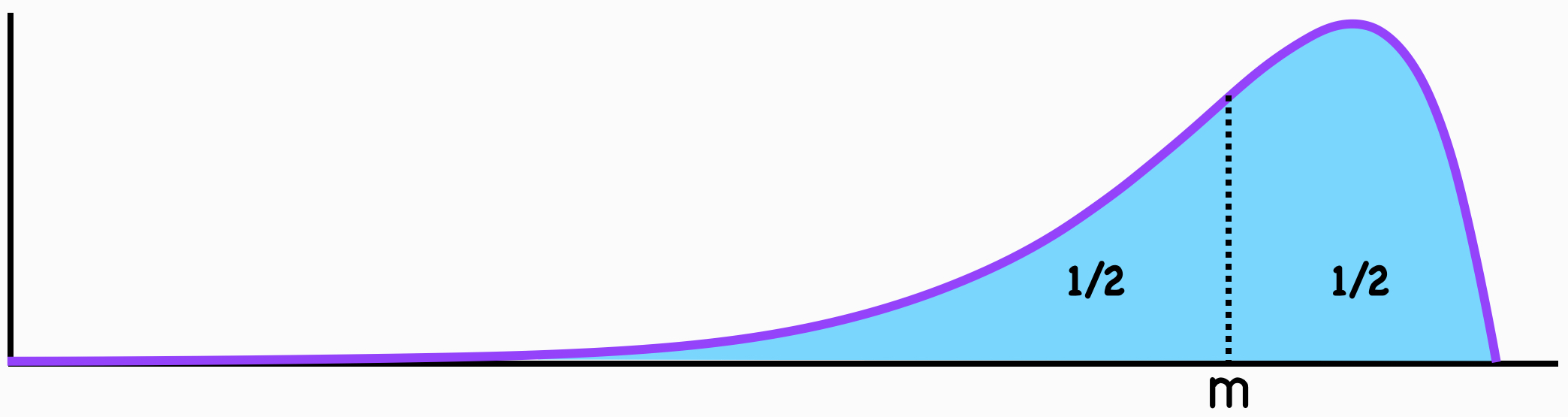As such, this question was intended to be just another CAS exercise, and so both trivial and pointless: push the button, write down the answer and on to the next question. The problem is, the median can also be determined by the equation

b)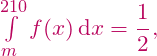or by the equation

c)And, since our function is only approximately a PDF, these three equations necessarily give three different answers: to the demanded two decimal places the answers are respectively 176.45, 176.43 and 176.44. Doh!

What to make of this? There are two obvious questions.

1. How did the VCAA end up with a PDF which isn’t a PDF?

It would be astonishing if all of the exam’s writers and checkers failed to notice the integral was not 1. It is even more astonishing if all the writers-checkers recognised and were comfortable with a non-PDF. Especially since the VCAA can be notoriously, absurdly fussy about the form and precision of answers (see below).

2. How was the error in 3(h)(ii) not detected?

It should have been routine for this mistake to have been detected and corrected with any decent vetting. Yes, we all make mistakes. Mistakes in very important exams, however, should not be so common, and the VCAA seems to make a habit of it.

OK, so the VCAA stuffed up. It happens. What happened next? That’s where the VCAA’s arrogance and cowardice shine bright for all to see. The one and only sentence in the Examiners’ Report that remotely addresses the error is:

“As [the] function f  is a close approximation of the [???] probability density function, answers to the nearest integer were accepted”.

The wording is clumsy, and no concession has been made that the best (and uniquely correct) answer is “The question is stuffed up”, but it seems that solutions to all of a), b) and c) above were accepted. The problem, however, isn’t with the grading of the question.

It is perhaps too much to expect an insufferably arrogant VCAA to apologise, to express anything approximating regret for yet another error. But how could the VCAA fail to understand the necessity of a clear and explicit acknowledgement of the error? Apart from demonstrating total gutlessness, it is fundamentally unprofessional. How are students and teachers, especially new teachers, supposed to read the exam question and report? How are students and teachers supposed to approach such questions in the future? Are they still expected to employ the precise definitions that they have learned? Or, are they supposed to now presume that near enough is good enough?

For a pompous finale, the Examiners’ Report follows up by snarking that, in writing the integral for the PDF, “The dx was often missing from students’ working”. One would have thought that the examiners might have dispensed with their finely honed prissiness for that one paragraph. But no. For some clowns it’s never the wrong time to whine about a missing dx.

UPDATE (16 June): In the comments below, Terry Mills has made the excellent point that the prior question on the exam is similarly problematic. 3(h)(i) asks students to calculate the mean of the probability distribution, which would normally be calculated as. For our non-PDF, however, we should should normalise by dividing by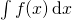. To the demanded two decimal places, that changes the answer from the Examiners’ Report’s 170.01 to 170.06.